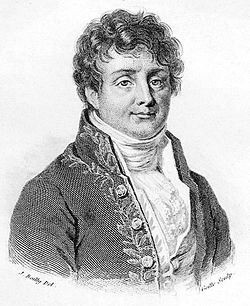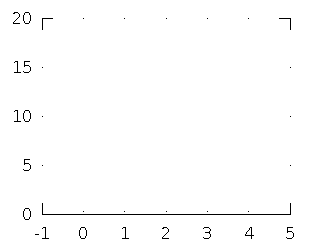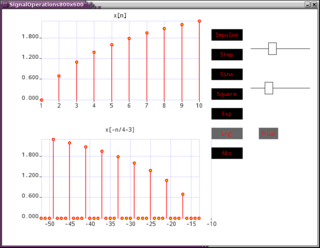# signals

## Fourier Reconstruction of an Image

Dec 11, 2014Any image can be reconstructed using just a superposition of plane waves of different spatial frequencies. This is a numerical experiment using a NumPy script that reconstructs an image one frequency component at a time.

Tags: math, signals, software

## Line Codes - Matlab / GNU Octave Code

Jul 19, 2012

I came up with the following approach to do line coding without for loops. My approach involves a bit of system theory, modulo 2 arithmetic, and sometimes just tricks founded on simple coincidence. So one is advised to be a little careful while sifting through the logic. But ultimately, the code is terse and fairly elegant.

Tags: signals, matlab, octave, software

## Convolution and Polynomial Multiplication

Mar 26, 2012

"Convolution in the time domain is multiplication in the frequency domain" is an oft-quoted statement. But the exact relation between the two is seldom stressed adequately. Mathematically, convolution and polynomial multiplication are one and the same process, as I shall explain shortly.

Tags: math, signals

## How does convolution work?

Mar 26, 2012The process of convolution works on the twin concepts of linearity and shift invariance. Here is an animated explanation of the concept.

Tags: math, signals

## Signal Operations Applet

Mar 17, 2012This is actually a set of two "applets" we did as part of an assignment for "Signals and Systems" in the fourth semester of college. The goal was to write an application which illustrated basic signal operations such as shifting, scaling, folding and also signal addition and multiplication.

Tags: signals, software